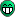# Crash From Robust fit

Hello All,

I am experiencing a crash when calling TGraph::Fit during a monte carlo. The crash seems to be random. It doesn’t fail on the same point or trial. The code included below works for many trials before failing. It gives the following error message followed by a seg fault and stack trace. Any help on this would be appreciated.

Error in <TMatrixTRow_const(const TMatrixT &,Int_t)>: row index out of bounds

*** Break *** segmentation violation
Generating stack trace…

[code]void scatter_analysis::run_tls_algorithm(int coin_in,const int n_points,Double_t energy_edges,Double_t results_x,Double_t results_y){

``````TString target={"&&coin_id<4&&coin_id!=2","&&(coin_id==4||coin_id==5||coin_id==7)","&&(coin_id==9||coin_id==11)","&&(coin_id==13||coin_id==15"};
TString cut = "En_gate==0&&psd_gate==1&&dbl_cut==0";

if(coin_in<16){
cut+="&&coin_id==";
cut+=coin_in;
}
else{
cut+=target[coin_in%4];
}

Double_t E1=1;
Double_t E2=energy_edges;

int nz = ptr_result_tree->Draw("ph_t:Ed",cut,"goff");
TGraph *g = new TGraph(nz,ptr_result_tree->GetV2(),ptr_result_tree->GetV1());

TF1 *fit =  new TF1("fit","pol0");

for(int i=0;i<n_points;i++){
g->Fit(fit,"ROB","goff",E1,E2);
results_x[i]=(E1+E2)/2;
results_y[i]=fit->GetParameter(0);
E1=E2;
E2=energy_edges[i+1];
}
delete g;
``````

}[/code]

if ((!ptr_result_tree) || (n_points < 0) || (n_points > 399)) return; // just a precaution
and right after the line:
int nz = …
if (nz < 2) return; // just a precaution
delete fit; // cleanup

Thanks for the feedback Wylie,

I added the lines, and am still getting the same crash. Do you have any idea what Matrix the error is referring to?

Cheers,

Josh

For the moment I don’t know where this TMatrixT comes from.
I can imagine that it’s related to some leaves of the “ptr_result_tree” which you use in the “Draw” expression or in the “cut” (i.e. one or more of “ph_t”, “Ed”, “coin_id”, “En_gate”, “psd_gate”, “dbl_cut”).

I noticed another problem: if you use the “B” fit option, you should initialize all parameters of the “fit” function (before calling g->Fit…):
fit->SetParameters(some_initial_value); // just one parameter for “pol0”

Hey Wilie,

The leaves of the tree are all Double_t. The crash doesn’t occur until I call fit on the TGraph.

I am not using the “b” option? The option specified is “ROB” which initializes the robust linear minimizer.

I was assuming the Matrix was internally generated by the fit function. Possibly the Error matrix? But the robust linear minimizer is not generating an error matrix…

Thanks,

Josh

Now I can see that you ask for “ROB” and not for “R0B”.Ok, so if I reduce the number of points I am passing to the fitter to 500, the code is totally stable.

Any thoughts?

What do you mean by “reduce the number of points I am passing to the fitter to 500”?

BTW. Just to be on the most safe side, you could try (though I don’t really believe that the number of selected entries exceeds “fEstimate” which is someting like 1000000 by default):
int nz = ptr_result_tree->Draw(“ph_t:Ed”, cut, “goff”);
if (nz > ptr_result_tree->GetEstimate()) nz = ptr_result_tree->GetEstimate();

Hey Wilie,

The range I am passing to the fitter is representative of some statistical bin size. Ie; the fitter is called for a subset of the data. When I select ranges that yield ~1000 points (changes a bit since this is a monte carlo) the program crashes at some random group of data points at a random trial. If I instead select ranges where the fitter looks at 500 data points, it no longer crashes.

Sadly nz is the total number of data points on the graph not the data points in the range.

Cheers,

Josh

I still don’t understand what you’re talking about.
The “scatter_analysis::run_tls_algorithm” has five “local” parameters (“coin_in”, “n_points”, “energy_edges”, “results_x”, “results_y”) and the only used “global” variable is the “ptr_result_tree”.
I hope you don’t try to set “n_points” to 500.

BTW. Basically, “nz” will be the number of “ptr_result_tree” entries that passed the “cut” (which you create in the “scatter_analysis::run_tls_algorithm”).